# Test: Electromagnetic & Analog Communications - 1

## 15 Questions MCQ Test GATE ECE (Electronics) 2023 Mock Test Series | Test: Electromagnetic & Analog Communications - 1

Description
Attempt Test: Electromagnetic & Analog Communications - 1 | 15 questions in 45 minutes | Mock test for Electronics and Communication Engineering (ECE) preparation | Free important questions MCQ to study GATE ECE (Electronics) 2023 Mock Test Series for Electronics and Communication Engineering (ECE) Exam | Download free PDF with solutions
QUESTION: 1

Solution:
QUESTION: 2

### An AC voltage source v = Vo sin cot is connected across a parallel plate capacitor 'C'. The displacement current in the capacitor is equal to

Solution:

Conduction current inThe connecting wires

= i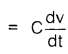= CωVo cos ωt   .............. (I)

For a parallel plate capacitor of area 'A and plate separation 'd',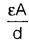......................(ii)

Electric field (E) =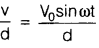Also,              D = Electric flux density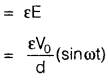Now, displacement current (id)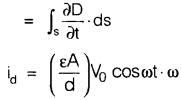= CωVo cos ωt   ............  (iii)

From equation (i) and (iii) we have

i = id

QUESTION: 3

### A plane wave is characterized by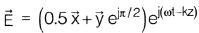The wave is

Solution:
QUESTION: 4

Skin depth at a frequency of 1.6 MHz inaluminium, σ = 38.2, μr = 1.

Solution:

Skin depth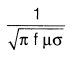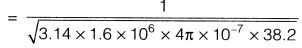= 64.4 mm

QUESTION: 5

An uniform sinusoidal plane wave in air hasthe following expression for electric field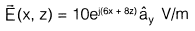The frequency of operation will be

Solution:

General expression,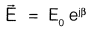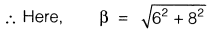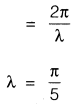Now, frequency =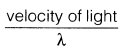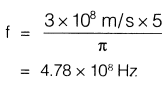QUESTION: 6

A dipole antenna was radiating with some excitation in free space radiating a certain amount of the power. If this antenna is immersed in a lake where water is non­magnetic and non-dissipative but has a dielectric constant of 81, the radiated power with the same excitation will

Solution:
QUESTION: 7

A dipole antenna of λ/8 length has anequivalent total loss resistance of 1.5ΩThe efficiency of the antenna is:

Solution:
QUESTION: 8

Match List-I (Laws) with List-II (Applica- tions) and select the correct answer:

List-I

A. Ampere's law

B. Biot's law

C. Coulomb's law

D. Gauss's law

List-II

To find the

1. force on a charge

2. force due to a current carrying

3. electric flux density at a point

4. magnetic flux density at a point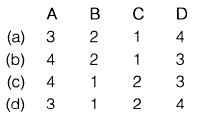Solution:
*Answer can only contain numeric values
QUESTION: 9

A signal m(t) is band limited upto 20 kHz is sampled at `fs' kHz. An ideal LPF having cut off frequency 57 kHz is used to reconstruct the m(t). The minimum value of `fs' required to reconstruct m(t) without distortion is,__________   kHz

Solution:

For the distortion less reconstruction of m(t)

signal,

fs - fm ≥ 57 kHz

⇒   fs ≥ 57 + fin
⇒   fs  ≥  77 kHz

QUESTION: 10

An AM transmitter is to deliver a total power of 100 W to an antenna. The percentage modulation is to be 100%. The power contained in each sideband is

Solution: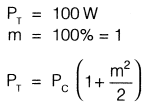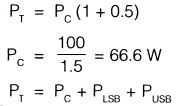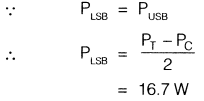QUESTION: 11

Which one of the following sequence is correct regarding bandwidth (BW) of various analog modulated system?

Solution:
QUESTION: 12

The nyquist sampling rate for the signal s(t) = 20 cos(50πt) . cos2(150πt) is,

Solution: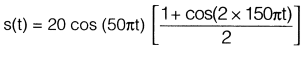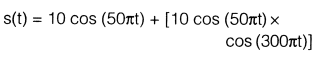The maximum frequency component will be

= 150 + 25

= 175 Hz

∴ Nyquist sampling rate

= fs

= 2 fm

= 2 x 175Hz

= 350 samples/sec

*Answer can only contain numeric values
QUESTION: 13

The modulation index of an FM signal having a carrier swing of 100 kHz and modulating frequency of 8 kHz is

Solution:

∴ Carrier swing = 2 x Δf

⇒  Δf = 50 kHz

Also, modulation index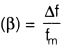∴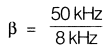= 6.25

QUESTION: 14

For the generation of an AM signal we have m(t) = cos (4π x 104 t) and c(t) = 5 cos(2π x 106 t) where m(t) represents message signal and c(t) represents carrier signal then what would be the relation between total power (PT) and power contained in one of its side band (Ps) if the modulation index is 0.5?

Solution:

∴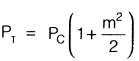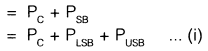Let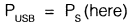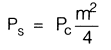⇒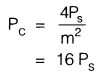.       .......(ii)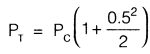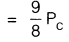∴ From equation (ii)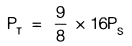⇒ PT = 18 Ps

QUESTION: 15

Percentage modulation of an AM wave having a power content of 8 kW at carrier frequency and 2 kW in each of its bands is given by

Solution:

Given That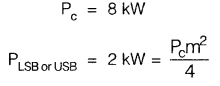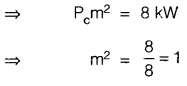⇒ m= 1

∴ % modulation = 100%Use Code STAYHOME200 and get INR 200 additional OFF Use Coupon Code